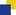International Journal of Scientific and Research Publications

# IJSRP Impact Factor:

IJSRP calculates impact factor of last year in fourth quarter of the consecutive year. For example impact factor of 2019 is calculated in 2020 4th quarter. Till 2015, IJSRP calculated impact factor based on actual citations received on published papers and from 2016 onwards we outsource to external agency and impact factor is calculated by SJIF. (WEBSITE: http://sjifactor.com/)

####Impact Factor 2020 : 7.662

Total Number of Citations for papers published in 2020 : 4507
* This impact factor is calculated by SJIF. Number of citation is calculated based on cited documents shown by Google Scholar, BASE and our other indexing partners for Year 2020. This impact factor is calculated based on citation (only) and not calculated by Thomson Reuters (ISI web of Science).

####Impact Factor 2019 : 6.97

Total Number of Citations for papers published in 2018 : 3097
* This impact factor is calculated by SJIF. Number of citation is calculated based on cited documents shown by Google Scholar, BASE and our other indexing partners for Year 2019. This impact factor is calculated based on citation (only) and not calculated by Thomson Reuters (ISI web of Science).

####Impact Factor 2018 : 6.64

Total Number of Citations for papers published in 2018 : 3047
* This impact factor is calculated by SJIF. Number of citation is calculated based on cited documents shown by Google Scholar, BASE and our other indexing partners for Year 2018. This impact factor is calculated based on citation (only) and not calculated by Thomson Reuters (ISI web of Science).

####Impact Factor 2017 : 6.14

Total Number of Citations for papers published in 2017 : 3086
* This impact factor is calculated by SJIF. Number of citation is calculated based on cited documents shown by Google Scholar, BASE and our other indexing partners for Year 2017. This impact factor is calculated based on citation (only) and not calculated by Thomson Reuters (ISI web of Science).

####Impact Factor 2016 : 4.41

Total Number of Citations for papers published in 2016 : 2851
* This impact factor is calculated by SJIF. Number of citation is calculated based on cited documents shown by Google Scholar, BASE and our other indexing partners for Year 2016. This impact factor is calculated based on citation (only) and not calculated by Thomson Reuters (ISI web of Science).

####Impact Factor 2015 : 3.27

Calculation Details:
Total Number of Citations for papers published in 2015 (A): 2799
Total Number of Published papers in 2015 (B): 856
Impact Factor (C): (A)/(B)

* This impact factor is calculated by SJIF. Number of citation is calculated based on cited documents shown by Google Scholar, BASE and our other indexing partners for Year 2015. This impact factor is calculated based on citation (only) and not calculated by Thomson Reuters (ISI web of Science).

####Impact Factor 2014 : 2.07

Calculation Details:
Total Number of Citations for papers published in 2014 (A): 1870
Total Number of Published papers in 2014 (B): 907
Impact Factor (C): (A)/(B)

* Number of citation is calculated based on cited documents shown by Google Scholar, BASE and our other indexing partners for Year 2014. This impact factor is calculated based on citation (only) and not calculated by Thomson Reuters (ISI web of Science).

####Impact Factor 2013 : 1.22

Calculation Details:
Total Number of Citations for papers published in 2013 (A): 1230
Total Number of Published papers in 2013 (B): 1007
Impact Factor (C): (A)/(B)

* Number of citation is calculated based on cited documents shown by Google Scholar, BASE and our other indexing partners for Year 2013.

####Impact Factor 2012 : 0.69

Calculation Details:
Total Number of Citations for papers published in 2012 (A): 506
Total Number of Published papers in 2012 (B): 734
Impact Factor (C): (A)/(B)

* Number of citation is calculated based on cited documents shown by Google Scholar and our other indexing partners for Year 2012.

####Impact Factor 2011 : 0.3

Calculation Details:
Total Number of Citations for papers published in 2011 (A): 4
Total Number of Published papers in 2011 (B): 12
Impact Factor (C): (A)/(B)

* Number of citation is calculated based on cited documents shown by Google Scholar and our other indexing partners for Year 2011.